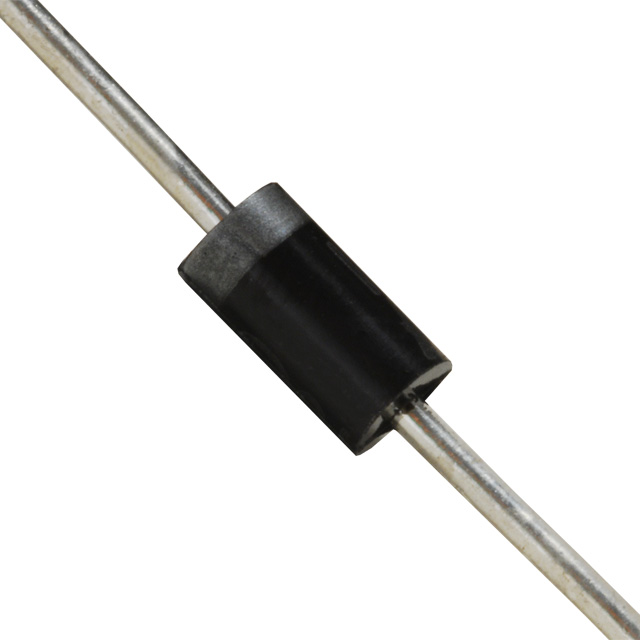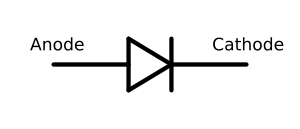Diode is a basic component that is fabricated from Semiconductor materials. It is a passive component since it does not have gain.

In the analysis of doping semiconductors, majority carriers for n-type and p-type semiconductors are free electrons and free holes respectively. A junction can be formed by joining n and p type of semiconductors. Such a P-N junction semiconductor device is known as diode.Structure or parts of diode have basic materials.They are two electrodes and a less conductive material. Between two electrodes, conductive material is kept. This material permits the current through it from one electrode to other but blocks the flow of current in reverse direction. The positive side of diode is anode and negative side is cathode. The positive side of power source is connected to anode of diode and negative side of power supply is connected cathode of diode.This kind of set up forward biases the diode with current flowing through it.

If connection is changed (+ve to -ve and vice versa), it reverse biases the diode by blocking the current in opposite direction. Diodes ever allow current through one direction and it is known as unidirectional current property of diodes.

A diode can be symbolished asVoltage – Current ( V-I) characteristics of diode is explained by Shockley diode equation given below.

##### I = Is ( eVd / ( nVt ) - 1)

where I is the diode current, Is is the reverse bias saturation current, Vd is the voltage across the diode, Vt is thermal voltage and n is emission co-efficient.